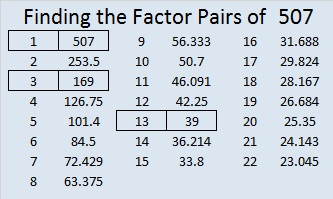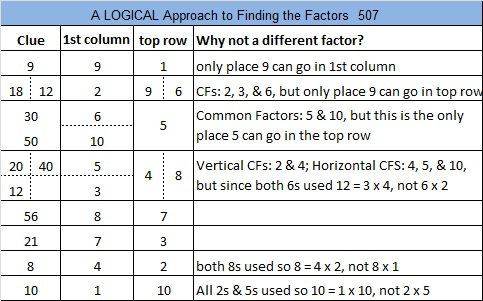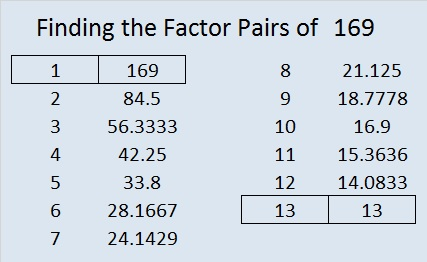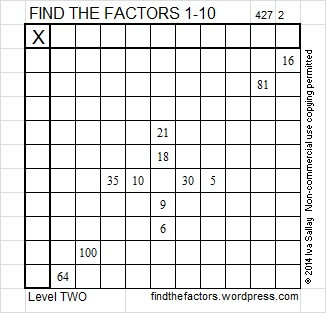# 507 and Level 6

507 cannot be evenly divided by 4 or 9, but to simplify its square root, I would still make a little cake:

If I didn’t recognize that 169 is a perfect square, I would apply some prime number divisibility tricks in numerical order on 169:

• 2: 169 is not even so it’s not divisible by 2.
• 3: 1 + 6 + 9 = 16 which is not a multiple of 3 so 169 is not divisible by 3.
• 5: The last digit is not 0 or 5, so 169 cannot be evenly divided by 5.
• 7: The difference between 16 and 9 x 2 is 2 which is not a divisible by 7, so 169 is not divisible by 7.
• 11: 1 6 + 9 = 4 which is not a multiple of 11, so 169 is not divisible by 11.
• 13: To check if 169 is divisible by 13, let’s make a second layer to our cake:Taking the square root of everything on the outside of the cake and multiplying it all together we get, √507 = (√3)(√13)(√13) = 13√3.

Here’s today’s puzzle:Print the puzzles or type the solution on this excel file: 10 Factors 2015-05-25

—————————————————————————————————

• 507 is a composite number.
• Prime factorization: 507 = 3 x 13 x 13, which can be written 507 = 3 x (13^2)
• The exponents in the prime factorization are 2 and 1. Adding one to each and multiplying we get (2 + 1)(1 + 1) = 3 x 2  = 6. Therefore 507 has exactly 6 factors.
• Factors of 503: 1, 3, 13, 39, 169, 507
• Factor pairs: 507 = 1 x 507, 3 x 169, or 13 x 39
• Taking the factor pair with the largest square number factor, we get √507 = (√169)(√3) = 13√3 ≈ 22.51666—————————————————————————————————# 169 and Level 2

 169 is a composite number and a perfect square. Factor pairs: 169 = 1 x 169 or 13 x 13 Factors of 169: 1, 13, 169 Prime factorization: 169 = 13 x 13 which can be written 13²—————————————————————–Excel file of puzzles and previous week’s factor solutions: 10 Factors 2014-07-07